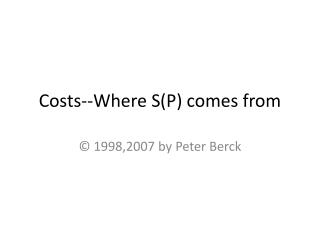# Costs--Where S(P) comes from - PowerPoint PPT PresentationDownload PresentationCosts--Where S(P) comes from

Costs--Where S(P) comes from
Download Presentation## Costs--Where S(P) comes from

- - - - - - - - - - - - - - - - - - - - - - - - - - - E N D - - - - - - - - - - - - - - - - - - - - - - - - - - -
##### Presentation Transcript

1. Costs--Where S(P) comes from © 1998,2007 by Peter Berck

2. The Cost Function C(q) • Output. Product firm sells • Input. Goods and services bought by firm and used to make output. • includes: capital, labor, materials, energy • C(q) is the least amount of money needed to buy inputs that will produce output q.

3. Fixed Costs • FC are fixed costs, the costs incurred even if there is no production. • FC = C(0). • Firm already owns capital and must pay for it • Firm has rented space and must pay rent

4. Average and Variable Costs • VC(q) are variable costs. VC(q) = C(q) - FC. • AC(q) is average cost. AC(q) = C(q)/q. • AVC is average variable cost. AVC(q) = VC(q)/q. • AFC is average fixed cost. AFC(q) = FC/q. • limits: AFC(0) infinity • and AFC(inf.) is zero.

5. AFC(Q) AFC Q

6. Marginal Cost • MC(q) is marginal cost. It is the cost of making the next unit given that Q units have already been produced • MC(q) is approximately C(q+1) - C(q). • Put the other way, C(q+1) is approximately C(q) + MC(q). • The cost of making q+1 units is the cost of making q units plus marginal cost at q.

7. C(Q) = Q2. A Diagram

8. Towards a better definition of MC • Per unit cost of an additional small number of units • Let t be the number of additional units • could be less than 1 • MC(q) approximately • {C(q+t) - C(q)}/t • MC(q) = limt0{C(q+t) - C(q)}/t

9. C q q+t MC: Slope of Tangent Line t C(q+t)-C(q)

10. C q q+t MC: Slope of Tangent Line

11. U Shaped Costs • Now let’s assume FC is not zero • AC(0) = AVC(0) + AFC(0) is unbounded • AC(infinity) = AVC(infinity) + 0 • Let’s assume MC (at least eventually) is increasing. • Fact: MC crosses AVC and AC at their minimum points

12. MC crosses AC at its minimum • Whenever AC is increasing, MC is above AC. multiply by q(q+1) and simplify

13. U Shaped Picture AC MC AVC \$/unit Q

14. Firm’s Output Choice • Firm Behavior assumption: • Firm’s choose output, q, to maximize their profits. • Pure Competition assumption: • Firm’s accept the market price as given and don’t believe their individual action will change it.

15. Theorem • Firm’s either produce nothing or produce a quantity for which MC(q) = p

16. Necessary and Sufficient • When Profits are maximized at a non zero q, P = MC(q) • P = MC(q) is necessary for profit maximization • P = MC(q) is not sufficient for profit maximization • (Is marijuana use necessary or sufficient for heroin use? Is milk necessary ….)

17. Candidates for Optimality p a 0 b Profits could be maximal at zero or at a “flat place” like a or b. Thus finding a flat place is not enough to ensure one has found a profit maximum

18. Discrete Approx. Algebra • Revenue = p q • p = p q - C(q) is profit • We will show (within the limits of discrete approximation) that “flat spots” in the p(q) function occur where p = MC(q)

19. Making one less unit • Now p(q*-1) - p(q*) = • { p (q*-1) - c(q*-1)}- { pq* - c(q*) } • = -p + [ c(q*) - c(q*-1) ] • = - p + mc(q*-1) • so -p + mc(q*-1) is the profit lost by making one unit less than q*

20. Making one more unit... • Now p(q*+1) - p(q*) = • { p (q*+1) - c(q*+1)}-[pq* - c(q*)] • = p + [ c(q*) - c(q*+1) ] • = p - mc(q*) • so p - mc(q*) is the profit made by making one more unit

21. Profit Max • If q* maximizes profits then profits can not go up when one more or one less unit is produced • so, p(q) must be “flat” at q* • No profit from one more: p - mc(q*)  0 • No profit from one less: - p + mc(q*-1)  0 • p- mc(q*-1)  0  p - mc(q*) • since mc increasing, p-mc must = 0 between • q*-1 and q* (actually happens at q*)

22. Picture and Talk MC \$/unit MC-P p P-MC q BIG q SMALL q*